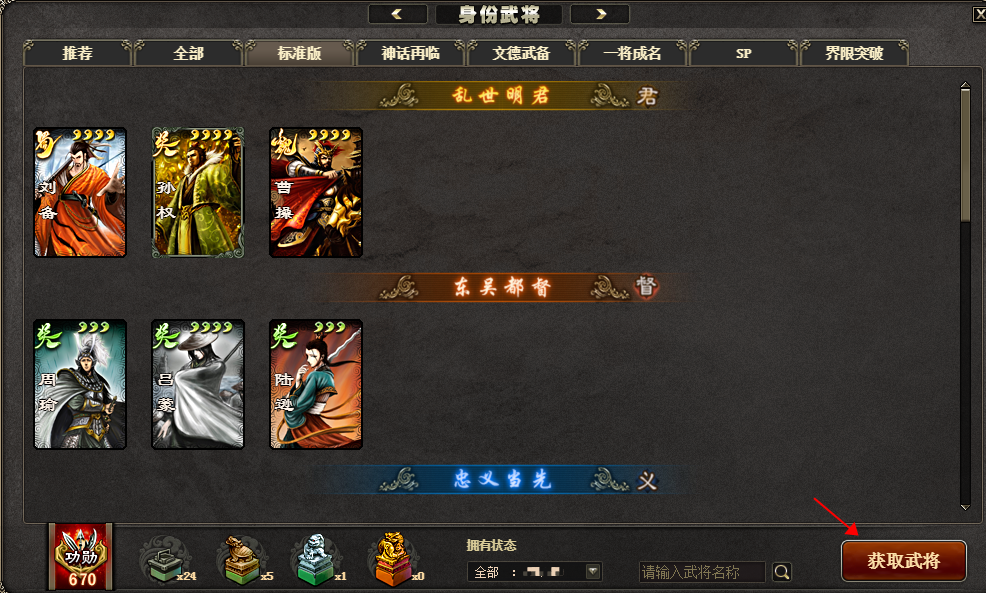[OL][活动]武将包全新上线

2021-01-20 17:21:09

三国杀OL新道具上线

1月20日版本更新后，玩家可在武将页面进行武将抽取。武将 重复返还灵宝经诰数量 概率 刘备(标) 关羽(标) 张飞(标) 诸葛亮(标) 赵云(标) 马超(标) 黄月英(标) 甘宁(标) 曹操(标) 吕布(标) 孙权(标) 周瑜(标) 大乔(标) 陆逊(标) 司马懿(标) 夏侯惇(标) 张辽(标) 许褚(标) 郭嘉(标) 甄姬(标) 吕蒙(标) 黄盖(标) 华佗(标) 貂蝉(标) 孙尚香(标) 黄忠(风) 魏延(风) 夏侯渊(风) 曹仁(风) 小乔(风) 周泰(风) 1 40.65% 典韦(火) 公孙瓒(SP) 于禁(将) 2 2.74% 庞德(火) 颜良文丑(火) 孙尚香(SP) 马谡(将) 3 2.74% 法正(将) 庞统(火) 太史慈(火) 袁绍(火) 孟获(林) 徐庶(将) 4 2.74% 荀彧(火) 卧龙诸葛(火) 徐晃(林) 曹丕(林) 孙坚(林) 祝融(林) 徐盛(将) 李通(SP) 马忠 庞德(SP) 马超(SP) 田丰(国战) 袁术(雷) 朱治(将5) 6 5.59% 华雄(标) 袁术(标) 马腾(国战) 纪灵(国战) 高顺(将) 7 2.03% 贾诩(林) 鲁肃(林) 董卓(林) 甘夫人(国战) 蒋琬费祎(国战) 关银屏(SP) 8 1.97% 吴国太(将) 潘凤(SP) 张辽(界限突破) 邓艾(山) 丁奉(SP) 曹仁(SP) 赵云(SP) 刘表(将2) 吴懿(将4) 郭嘉(界限突破) 公孙瓒(界限突破) 9 4.07% 刘备(界限突破) 黄月英(SP) 貂蝉(SP) 张飞(界限突破) 步骘(SP) 凌统(将) 曹操(界限突破) 贾诩(SP) 文聘(SP) 刘禅(山) 曹彰(将2) 程普(将2) 甘宁(界限突破) 公孙渊(将5) 朱然(将3) 华雄(界限突破) 孔融(国战) 关羽(SP) 张郃(山) 曹洪(SP) 陈宫(将) 廖化(将2) 韩当(将2) 张春华(将) 张嶷(将5) 顾雍(将4) 韩浩史涣(将4) 10 8.13% 吕布(界限突破) 关羽(界限突破) 姜维(山) 孙策(山) 蔡文姬(山) 张昭张纮(山) 赵云(界限突破) 黄盖(界限突破) 司马懿(界限突破) 曹植(将) 步练师(将2) 伏完(SP) 马良(SP) 关兴张苞(将2) 吕蒙(界限突破) 李典(界限突破) 朱桓(将4) 夏侯霸(SP) 钟会(将2) 郭淮(将3) 大乔(界限突破) 夏侯惇(界限突破) 华佗(界限突破) 沮授(将4) 蔡夫人(将4) 王异(将2) 11 6.66% 徐氏(原创7) 何太后(SP) 张松(将4) 曹叡(将5) 张鲁(SP) 司马朗(SP) 王基 曹节(原创7) 嵇康(原创7) 于吉(界限突破) 孙休(将5) 孙亮(阴) 吕虔 诸葛亮(界限突破) 夏侯氏(将5) 阚泽 戏志才 卑弥呼 郭皇后(原创6) 董白(SP) 孙登(原创6) 岑昏(原创6) 张让(原创6) 吴苋(原创7) 18 4.57% 糜竺(SP) 曹昂(SP) 马超(界限突破) 祖茂(SP) 曹真(将4) 周仓(将4) 麹义 刘协(SP) 陈群(将4) 蔡文姬(SP) 徐庶(界限突破) 许褚(界限突破) 全琮(将5) 刘虞(原创6) 黄皓(原创6) 臧霸(国战) 糜夫人(国战) 陈武董袭(国战) 蒋钦(国战) 诸葛瑾(SP) 荀攸(将2) 12 5.08% 诸葛诞(SP) 新张角(风) 刘封(将3) 马岱(将2) 新于吉(风) 周瑜(界限突破) 潘璋马忠(将3) 虞翻(将3) 陆逊(界限突破) 关平(将3) 姜维(SP) 伏皇后(将3) 孙鲁班(将4) 邹氏(国战) 严颜(阴) 13 3.56% 陈到(雷) 31 0.18% 辛宪英(原创7) 薛综(原创7) 蔡邕(原创7) 王平(阴) 曹冲(将3) 甄姬(界限突破) 满宠(将3) 李儒(将3) 严白虎(SP) 张任(国战) 刘谌(将5) 董允 曹休(将5) 钟繇(将5) 郭图逢纪(将5) 贺齐 孙乾 鲁芝 李严(原创6) 孙资刘放(原创6) 14 5.08% 马云騄(SP) 张角(界限突破) 38 0.18% 简雍(将3) 貂蝉(界限突破) 乐进(SP) 15 0.71% 秦宓(原创7) 刘琦 16 0.41% 张星彩(SP) 黄月英(界限突破) 王粲 17 0.61% 张济(SP) 大乔小乔(SP) 24 0.25% 左慈(山) 59 0.05% 郝昭(雷) 张绣(雷) 陆绩(阴) 许攸(阴) 周妃(雷) 毌丘俭(雷) 陆抗(雷) 苏飞(SP) 216 0.12% 吕范(国战) 审配 19 0.25% 孙鲁育(SP) 22 0.12% 庞统(界限突破) 小乔(界限突破) 太史慈(界限突破) 36 0.17% 李傕郭汜(国战) 27 0.10% 荀谌 37 0.08% 刘禅(界限突破) 夏侯渊(界限突破) 董卓(界限突破) 何进(新1v1) 牛金(新1v1) 韩遂(新1v1) 许靖 60 0.30% 李傕(SP) 郭汜(SP) 243 0.04% 魏延(界限突破) 庞德(界限突破) 67 0.08% 孙策(界限突破) 72 0.05% 兀突骨 蹋顿(SP) 周鲂(SP) 张辽(SP) 120 0.09% 卢植(阴) SP庞统 高览 150 0.07% 诸葛恪(SP) 张宝(SP) 蒯良蒯越(阴) 诸葛瞻(雷) 蒋干(SP) 162 0.09% 卧龙诸葛亮(界限突破) 34 0.10% 张昌蒲 170 0.04% 黄权(SP) 唐咨(SP) 189 0.05% 袁绍(界限突破) 66 0.07% 孙权(界限突破) 270 0.04% 杨修(SP) 陈琳(SP) 孙皓(SP) 336 0.05% 程昱(SP) 360 0.04% 灵雎(SP) 384 0.04% 士燮 1500 0.005% 司马徽 1600 0.005%

2021-01-20

•相当给力

48

•精品文章

6

•老夫惊了

355

•囧了一下

54

•这不科学

57

•路过一下

8